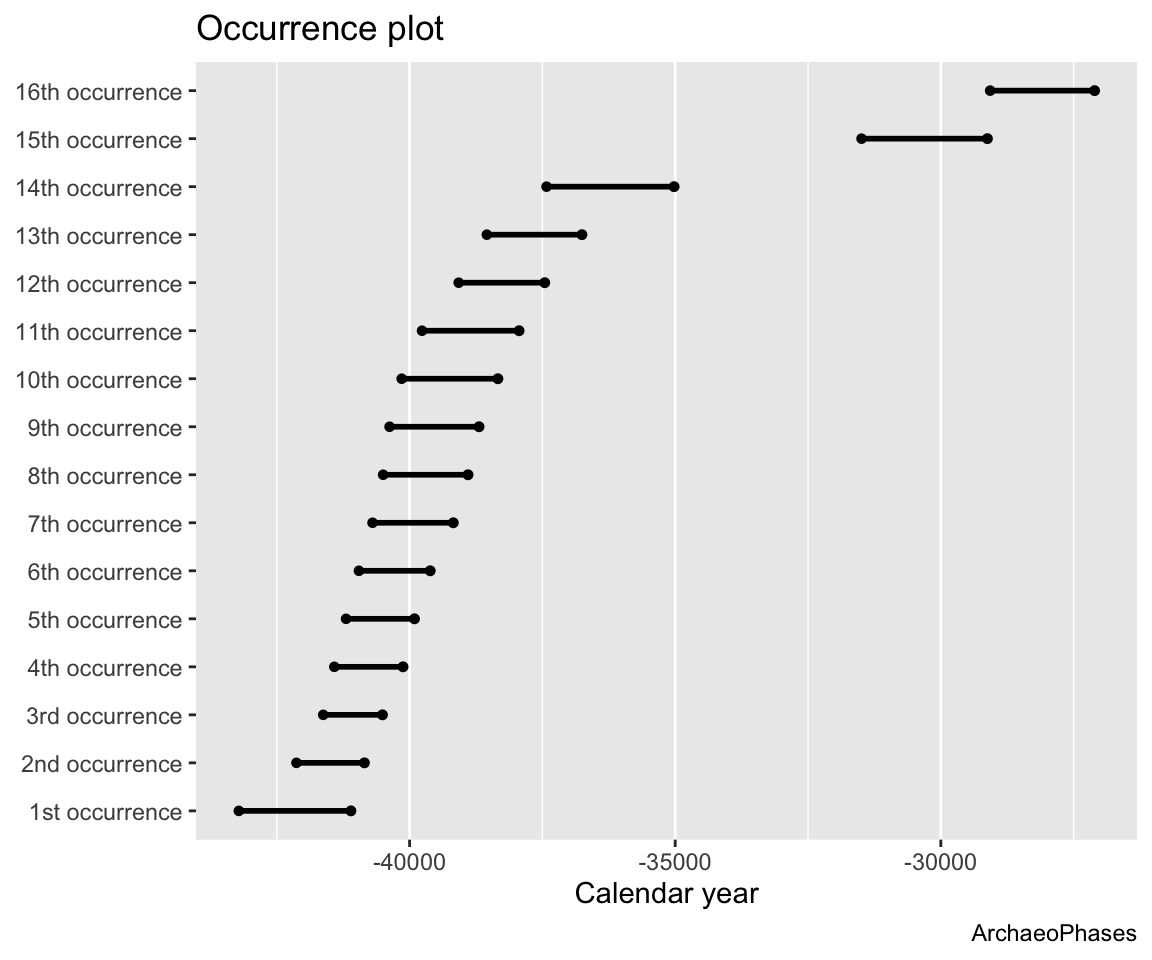# 2. ArchaeoPhases : Analysis of a series of dates

## Analysis of a series of dates

### Tempo Plot

The tempo plot has been introduced by Thomas S. Dye (Dye, T.S. (2016) Long-term rhythms in the development of Hawaiian social stratification. Journal of Archaeological Science, 71, 1–9). See Philippe and Vibet 2017 for more statistical details.

The tempo plot is one way to measure change over time: it estimates the cumulative occurrence of archaeological events in a Bayesian calibration. The tempo plot yields a graphic where the slope of the plot directly reflects the pace of change: a period of rapid change yields a steep slope and a period of slow change yields a gentle slope. When there is no change, the plot is horizontal. When change is instantaneous, the plot is vertical.

The code is the following (Warning : be patient. The execution time depends on the number of dates included.)

data("KADatesChronoModel")
tempo_plot(KADatesChronoModel, c(2:10), level = 0.95)
NULL
<0 rows> (or 0-length row.names)

From these graphs, we can see that the highest part of the sampled activity is dated between -45 000 to -35 000 but two dates are younger, at about -32 000 and -28 000.

### Tempo Activity Plot

The activity plot displays the derivative of the Bayes estimate of the Tempo plot. It is an other way to see changes over time.

The code is the following (Warning : be patient. The execution time depends on the number of dates included.)

tempo_activity_plot(KADatesChronoModel, c(2:10))
          t y
1 -41962.14 0
2 -41924.28 0
[ reached 'max' / getOption("max.print") -- omitted 447 rows ]

### Occurrence Plot

The Occurrence plot calculates the calendar date t corresponding to the smallest date such that the number of events observed before t is equal to k, for k =[(1, 16)]. The Occurrence plot draws the credible intervals or the highest posterior density (HPD) region of those dates associated to a desired level of confidence.

data("KADatesChronoModel")
OccurrencePlot(KADatesChronoModel, c(2:17), level = 0.95, newWindow= FALSE)
Registered S3 methods overwritten by 'ggalt':
method                  from
grid.draw.absoluteGrob  ggplot2
grobHeight.absoluteGrob ggplot2
grobWidth.absoluteGrob  ggplot2
grobX.absoluteGrob      ggplot2
grobY.absoluteGrob      ggplot2
Warning: Use of Ordered.df$y.labs is discouraged. Use y.labs instead. Use of Ordered.df$y.labs is discouraged. Use y.labs instead.